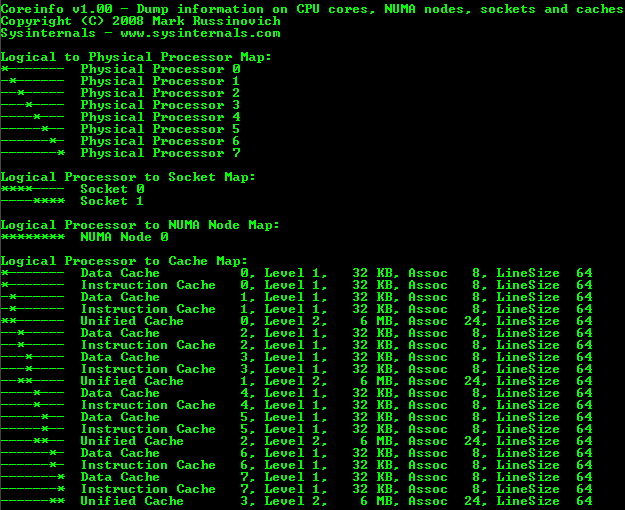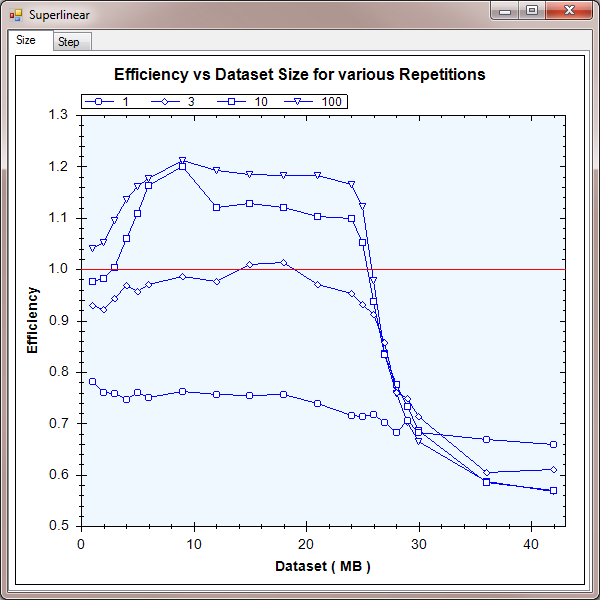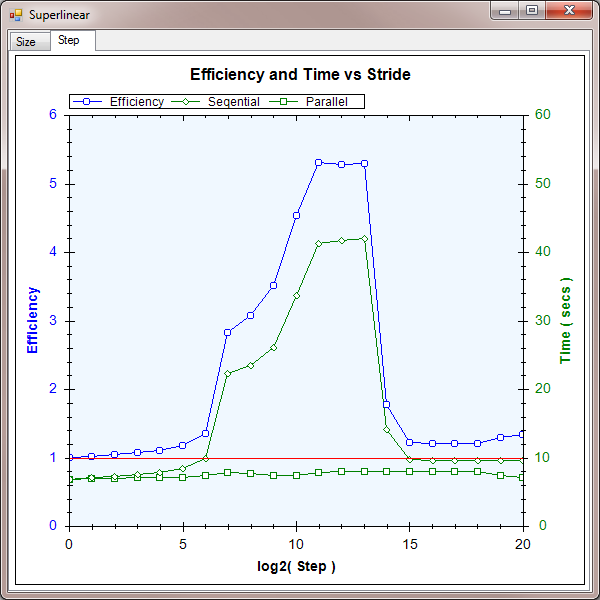## Superlinear: an investigation into concurrent speedup

By Nicholas ButlerPublicly recommend on Google

## Introduction

This is a short article showing superlinear speedup of a memory-bound algorithm running on an 8-core machine with 24 MB of level 2 cache.

This is an article of three halves. The first half describes the metrics I will be using. The second half shows a common result and the third half investigates just how much speedup is possible in a best case.

The best result I achieved is an efficiency of 5 times. So instead of running 8 times faster on an 8-core machine, the parallel implementation runs 40 times faster.

## Background

#### Definitions

Firstly, here is a quick recap of some definitions.

speedup = sequential time / concurrent time

So if you have a 4-core box that runs an algorithm sequentially in 8 seconds and in parallel in 2 seconds, you have a speedup of 4. If you then run the algorithm on an 8-core box and achieve a time of 1 second, you have a speedup of 8.

The example above is called linear speedup. This is normally the target when you convert to a parallel algorithm: run on a 4-core box and it goes 4 times faster; run on an 8-core box and it goes 8 times faster; and so on.

Normally, you don't achieve this. You might achieve 2.5 seconds on a 4-core box or 1.25 seconds on an 8-core box. These times are speedups of 3.2 and 6.4 respectively.

efficiency = speedup / number of cores

When comparing 3.2 and 6.4, it's not obvious how well your concurrent algorithm is scaling on a bigger machine, so we introduce the normalized metric: efficiency. The example gives an efficiency of 0.80 = 80% in both cases. So you could immediately say that although you are not achieving linear efficiency, your algorithm is scaling well.

Efficiencies are grouped into 3 possibilities. Efficiencies less than 1 are called sublinear. This is the most common case. An efficiency of exactly 1 is called linear. And the holy grail ( and the subject of this article ) is efficiencies greater than 1, called superlinear.

1-core 4-core 8-core efficiency
time speedup time speedup time speedup percent category
8 1 2.5 3.2 1.25 6.4 80% sublinear
8 1 2 4 1 8 100% linear
8 1 1.6 5 0.8 10 125% superlinear
comparison of speedup and efficiency

This article shows that considerable superlinear efficiencies are possible in some cases.

#### How is superlinear efficiency possible?

Since any concurrent algorithm can be rewritten as a sequential one, surely it is impossible to achieve superlinear speedup?

Well, this article proves that it is possible! If your algorithm is limited by the resources available to a single core, then adding cores and having each core work on a smaller set of data can make your machine disproportionately faster.

Basically, by using more of the resources in your machine, you can run your code more efficiently in some cases.

#### Memory architecture

For this example, I have targeted the level 2 cache in my dual-processor quad-core Xeon box. So the first step was to find out exactly what hardware was present.

Joe Duffy, author of "Concurrent Programming on Windows" and lead developer of the .NET 4.0 Parallel Extensions team, wrote a utility to do exactly that last year. He gave it[^] to Mark Russinovich who added it to the SysInternals toolset as CoreInfo[^]. Here is the output for my machine:coreinfo output

From this you can see, in quite some detail, the memory architecture present in your machine. I have extracted the info relevant to this article to produce the diagram below:simplified memory architecture

Each core has access to 6 MB of level 2 cache, shared between a pair of cores. This gives a total of 24 MB of cache present.

## Part I: Dataset size

I wanted to show that as the dataset increased beyond the 6 MB available to a single core, the concurrent implementation would begin to outperform the sequential implementation with superlinear speedups. This happens because when the dataset is larger than the cache, the CPUs have to access main memory, which takes hundreds of clock cycles. Also, as the total 24 MB limit was passed, both implementations would suffer cache misses and the efficiency would drop.

The code is very simple. The dataset is a `byte[]` called `counters` of varying length. The 'work' is just incrementing every element in the array. That's it! Here is a code snippet:

```for ( int n = 0 ; n < REPEAT ; n++ )
for ( int i = 0 ; i < length ; i++ )
counter[ start + length ]++;```

For the sequential runs, `start` = 0 and `length` = the size of the array. For the concurrent runs, the array is split into equal ranges and the parameters are adjusted accordingly for each thread. The other parameter is `REPEAT`. This just repeats the increment loop a given number of times.

On the first run of the increment loop, it doesn't matter how much cache is used because all the data must be loaded from main memory. These are called "compulsory misses". However, on subsequent runs, the concurrent implementation shows marked efficiency gains due to the larger cache available which reduces "capacity misses".

Here is the graph of the results:Part I results

When `REPEAT` = 1, the concurrent implementation has no special advantage over the sequential implementation, but does suffer from the usual overheads. This leads to a fairly steady efficiency of around 70% - 80%. Remember, this still means that we see a speedup of around 6 times on an 8-core machine. It's just sublinear.

As `REPEAT` increases, we see the efficiency gains I was looking for. For `REPEAT` = 100, the efficiency was between 110% - 120% for a broad range of dataset sizes from 3 MB to 24 MB. If you're tuning your application for performance, this is a very valuable boost. It means your code can run nearly 10 times faster on an 8-core machine.

As the dataset size increases beyond the 24 MB total cache present in the machine, the concurrent implementation also suffers cache misses and efficiency drops down dramatically to quite low values around 60%. This is half the peak efficiency and shows just how important the cache can be.

## Part II : Stride

While the results in part I above would be more than welcome in a real world application, I wondered just how high efficiency can become. So in this part, I mess up memory locality as much as possible. The results are difficult to predict, but show a maximum efficiency of 500%. On an 8-core machine, this equates to a 40 times speedup!

I chose a dataset size of 24 MB and `REPEAT` = 10 for this test. Then, instead of incrementing the array in order, I iterate over it in strides. This is the calculation of which index to increment in the loop:

```for ( int step = 0 ; step <= 20 ; step++ )
for ( int n = 0 ; n < REPEAT ; n++ )
for ( long i = 0 ; i < length ; i++ )
{
long prod = i << step;
long mod = prod % length;
long div = prod / length;
long index = start + mod + div;

counter[ index ]++;
}```

So the array is accessed with indices following this pattern:

Iteration Linear Stride
0 0 0
1 1 16
2 2 32
3 3 48
... ... ...
n n 1
n+1 n+1 17
n+2 n+2 33
n+3 n+3 49
... ... ...

This is very bad for locality, but it should only matter for the sequential implementation as the dataset can fit into the total L2 cache available to the concurrent implementation.

Here is a graph of the results. I have shown the times for the sequential and parallel implementations, as well as the efficiency calculated from these values.Part II results

As you can see, the times for the sequential implementation increase dramatically for strides between 27 and 213. The times for the concurrent implementation remain fairly constant, as expected, giving efficiencies up to 5 = 500%. If your application happens to fall into the sweet spot, this shows that you can make spectacular performance gains by considering your usage of cache.

I was surprised that the times for the sequential implementation became better for strides above 213. I suspect this is something to do with the associativity policy, but I have not confirmed that. If you get to the root of this, please leave a comment in the forum below - I would be very interested.

## Conclusion

Well, that's about it. In this article I have shown efficiencies up to 500%, which means a 40 times speedup on an 8-core machine. If you have performance problems, it is worth looking at memory locality - both temporal and spatial.

## History

• 2009 - 10 - 11: First version.